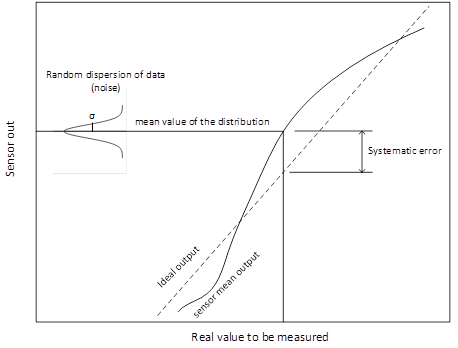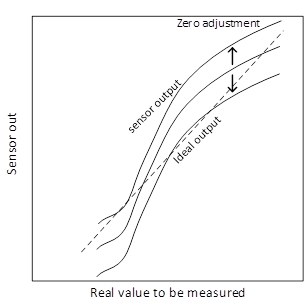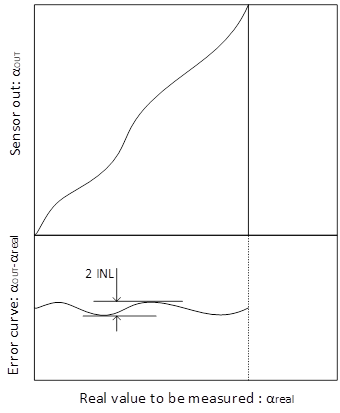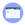## Accuracy and INL

The accuracy is defined as the maximum systematic error over the sensor range. The systematic error is the difference between the sensor mean output and the real value to be measured.For angle sensors, the zero angle indication can often be changed arbitrarily and adjusted by the user.To characterize an angle sensor system, one must know how flat the “error curve” is. The error curve is the difference between the sensor mean output and a reference angle usually given by an accurate encoder, regardless of the zero setting.The integral nonlinearity (INL) is a parameter that specifies the maximum deviation between the device output and a linear fit of the output. The INL is defined by:

$$INL={1 \over2} (max⁡(error curve)-min⁡(error curve) )$$.